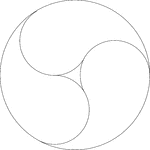# Extreme Yin-Yang

Geometry Level 5The figure above is created by three tear drop shaped figures. If the large circle has a radius of 9 units, find the maximum possible area of the figure located at the circle's center.

Assume:

• The tear drops have circular heads.

• You can change the size of the tear drops so that one is bigger than the others.

• You may use a calculator for operations such as +, -, /, $*$.

×